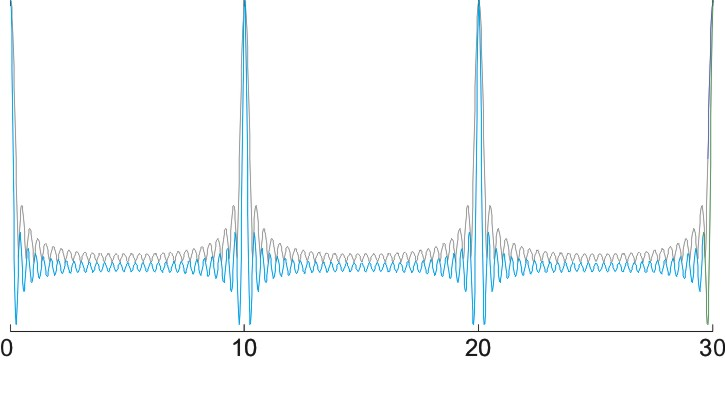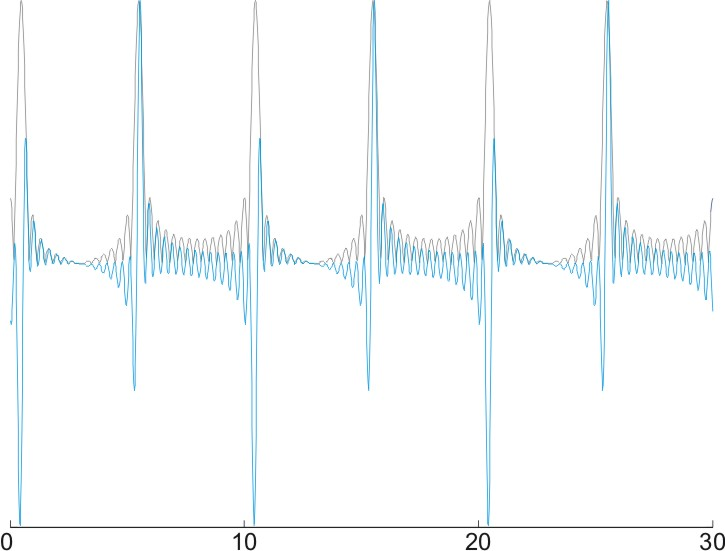# Periodicity of Sounds and of Envelopes

Pitch is determined in most cases by the periodicity of the sound waveform. However, some sounds have other, more subtle periodicities. In some cases, these periodicities may determine the pitch, but in other cases they don't. Here such subtle periodicity is illustrated - the periodicity of the envelope.

Look at the figure below. This sound (blue) was generated as a sum of many harmonics in 'cosine-phase' - this is a complicated way to say that all harmonics peak together at the beginning of the pitch period. The resulting waveform is very peaky, but has fast fluctuations which are smallest at the midpoint between two peaks and which increase in size around the peaks. One could imaging an 'envelope' - a positive waveform that would measure the overall energy of the sound waveform at each moment in time. The envelope (here computed using the 'Hilbert transform' - this is a side issue here) is plotted in gray.Sound

Envelope

Now, observe the figure below. This sound was generated with the same harmonics, except that only every other harmonic is in cosine-phase. The other half are in 'sine-phase' - this means that instead of peaking at the beginning of the pitch period, they have an upward zero crossing there (remember, harmonics are sine waves!). In contrast with the previous sound, this one has two 'events', with similar shape but opposite polarity, during the pitch period. Its periodicity is nevertheless exactly that of the previous sound, and the evoked pitch is the same. However, the envelope, which measures the overall energy at each moment in time, has now two peaks within each pitch period, and in fact, when using the same method for computing it, has half the period of the waveform. Thus, it has a pitch which is twice as high (200 Hz instead of 100 Hz in this case).Sound

Envelope

This is important when we study the responses of neurons to periodic sounds. Neurons may well respond to the envelope, rather than to the sound itself. Such neurons cannot encode pitch, even when they are sensitive to periodicity, because they do not give the right answer for alternate-phase harmonic complexes.